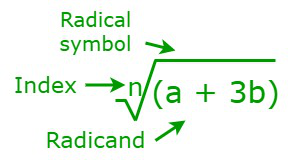Open in App
Not now

• Last Updated : 03 Jul, 2022

In mathematics, an expression with a root is referred to as a radical. A radical can be used to describe various types of roots of a number, including square roots, cube roots, fourth roots, etc. For example, common radicals like the square root and cube root are expressed by the symbols √ and ³√, respectively, where “3” is the degree or index of the number. If the index of the radical is not mentioned, then it is considered a square root. The word “Radical” is extracted from the Latin word “radix” which means “Root”. Let us consider an example to understand the concept of radical better.In the figure shown above, “n” is the index of the radical, “(a + 3b)” is the radicand, and “(n√)” is the radical symbol, and it is symbolically written as “nth root of (a+3b).” The index of a radical helps to determine how many times a number must be multiplied by itself in order to equal a radicand. And also an index of a radical is regarded as an antithesis of an exponent.

### General rules of a Radical Formula

The following are some general guidelines for radicals.

• The resultant will be positive if there is a positive number under the radical.
• The resultant will be negative if the number under the radical is negative. Take note radical negative numbers are only calculated if their index is odd.
• If the index of the radical is not mentioned, then it is considered a square root.
• Multiplication of numbers applies to numbers that share the same radical and index. For example, 5√21 × 5√15 =  5√(21×15) =  5√315.
• Similarly, the division is also applicable to numbers that have the same radical. For example, ³√25/³√5 = ³√(25/5) = ³√5.
• The reverse of the multiplication can be applied by splitting the number under the same radical. For example, √32 = √16 × √2 = 4√2.
• In any equation, a radical can be expressed in its exponential form.
• An index number’s inverse exponent is equivalent to the radical itself.

n√(x) is a radical expression of the “nth root of x”. A radical expression is said to be simplified, it has to be radically free. So, for making the given expression radical free, we need to power both sides of the given equation with “n”.

n√(x) = p

(x)1/n = p

(x1/n)n = (p)n

x = (p)n

n is the index of the radical, and

(n√) is the radical symbol or nth root.

### Sample Problems

Problem 1: Solve the radical, √y = 11, using the radical formula.

Solution:

Given,

√y = 11

(y)1/2 = 11

Now squaring on both sides we get

⇒ [(y)1/2]2 = (11)2

⇒ y = (11)2 ⇒ y = 121

Hence, the value of y is 121.

Problem 2: Solve the radical expression (7 + 5√a)/b, where a = 36 and b = 4.

Solution:

Given,

a = 36 and b = 4

By substituting the values of a and b in the given radical expression we get

(7 + 5√a)/b

= (7 + 5√36)/4

= (7 + 5 × 6)/4

= 37/4 = 9.25

Hence, the value of the given radical expression is 9.25.

Problem 3: Simplify √(175a4b5)/√(7b).

Solution:

√(175a4b5)/√(7b)

By using the quotient rule, we get

== √(25a4b4)

= 5a2b2

Hence, the value of the given radical expression is 5a2b2.

Problem 4: Solve √(3x+9) − 6 = 0

Solution:

Given,

√(3x+9) − 6 = 0

⇒ √(3x+9) = 6

Now squaring on both sides we get

⇒ (3x + 9) = (6)2

⇒ 3x + 9 = 36

⇒ 3x = 36 – 9 = 27

⇒ x = 27/3 = 9

Hence, the value of x is 9.

Problem 5: Simplify ³√36a5b2 ³√6ab.

Solution:

Given,

³√36a5b2 ³√6ab

= ³√(36a5b2) × (6ab)

=  ³√(216a6b3)

= ³√(63a6b3)

= 6a2b

Hence, the value of the given radical expression is 6a2b.

Problem 6: Find the value of 3/(2+√5).

Solution:

Given,

Now, multiply and divide the given term with (2 – √5)

= 3/(2 + √5) × (2 – √5)/(2- √5)

= 3(2 – √5)/(22– 5)     {Since, (a + b)(a – b) = a2 – b2}

= 3(2 – √5)/(4 – 5)

= 3(2 -√5)/(-1)

= 3(√5 – 2)

Hence, 3/(2 + √5) = 3(√5 – 2).

My Personal Notes arrow_drop_up
Related Articles